# Detect Cycle in an Undirected Graph (using DFS)

Problem Statement: Given an undirected graph with V vertices and E edges, check whether it contains any cycle or not.

Examples:

```Example 1:
Input:
V = 8, E = 7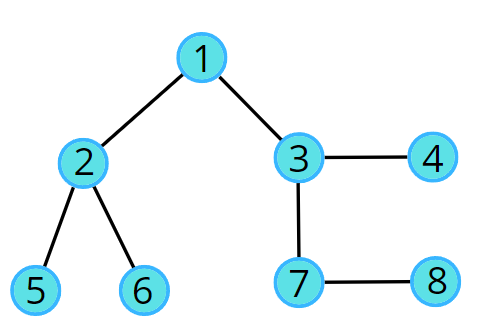Output: No

Explanation: No cycle in the given graph.
```
```Example 2:
Input:
V = 8, E = 6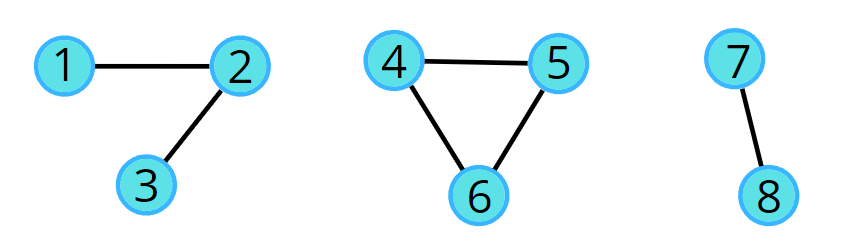Output: Yes

Explanation:
4->5->6->4 is a cycle.
```

### Solution

Disclaimer: Don’t jump directly to the solution, try it out yourself first.

### Intuition:

The cycle in a graph starts from a node and ends at the same node. DFS is a traversal technique that involves the idea of recursion and backtracking. DFS goes in-depth, i.e., traverses all nodes by going ahead, and when there are no further nodes to traverse in the current path, then it backtracks on the same path and traverses other unvisited nodes. The intuition is that we start from a source and go in-depth, and reach any node that has been previously visited in the past; it means there’s a cycle.

### Approach:

The algorithm steps are as follows:

• In the DFS function call make sure to store the parent data along with the source node, create a visited array, and initialize to 0. The parent is stored so that while checking for re-visited nodes, we don’t check for parents.
• We run through all the unvisited adjacent nodes using an adjacency list and call the recursive dfs function. Also, mark the node as visited.
• If we come across a node that is already marked as visited and is not a parent node, then keep on returning true indicating the presence of a cycle; otherwise return false after all the adjacent nodes have been checked and we did not find a cycle.

NOTE: We can call it a cycle only if the already visited node is a non-parent node because we cannot say we came to a node that was previously the parent node.

For example, node 2 has two adjacent nodes 1 and 5. 1 is already visited but it is the parent node ( DFS(2, 1) ), So this cannot be called a cycle.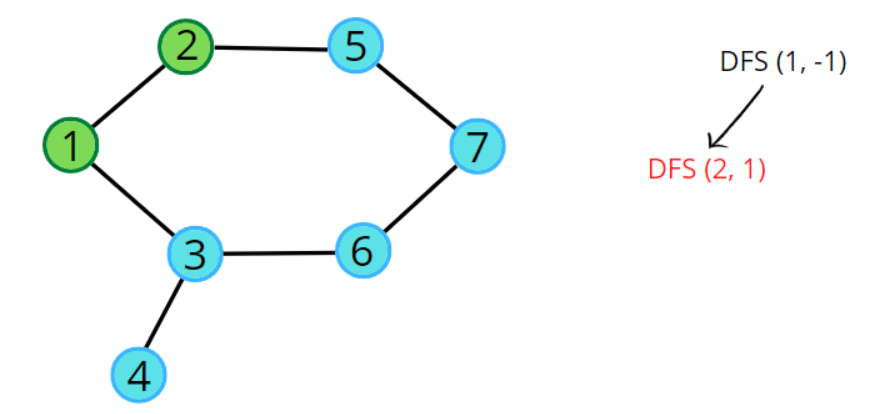Node 3 has three adjacent nodes, where 4 and 6 are already visited but node 1 is not visited by node 3, but it’s already marked as visited and is a non-parent node ( DFS(3, 6) ), indicating the presence of cycle.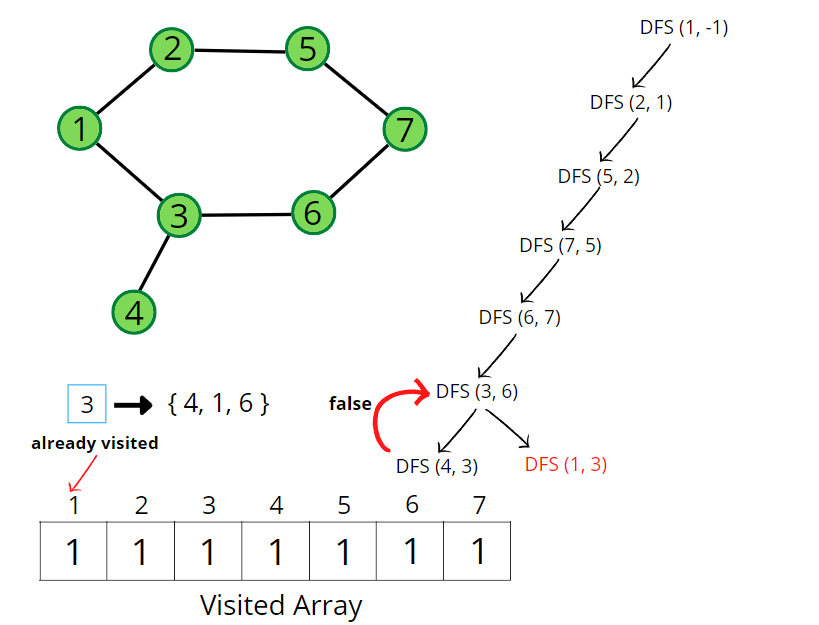Pseudocode: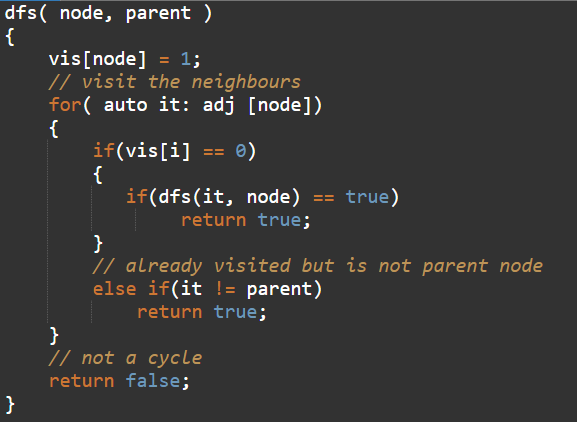A graph can have connected components as well. In such cases, if any component forms a cycle then the graph is said to have a cycle. We can follow the algorithm for the same: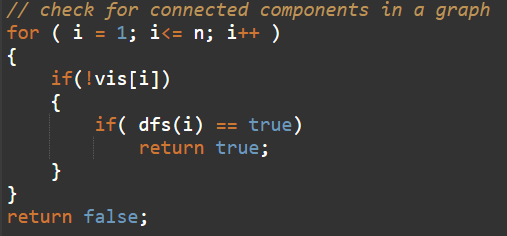Consider the following graph and its adjacency list.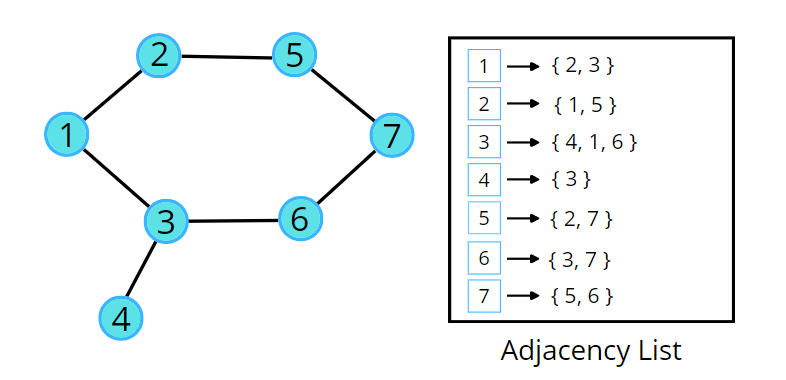Consider the following illustration to understand the process of detecting a cycle using DFS traversal.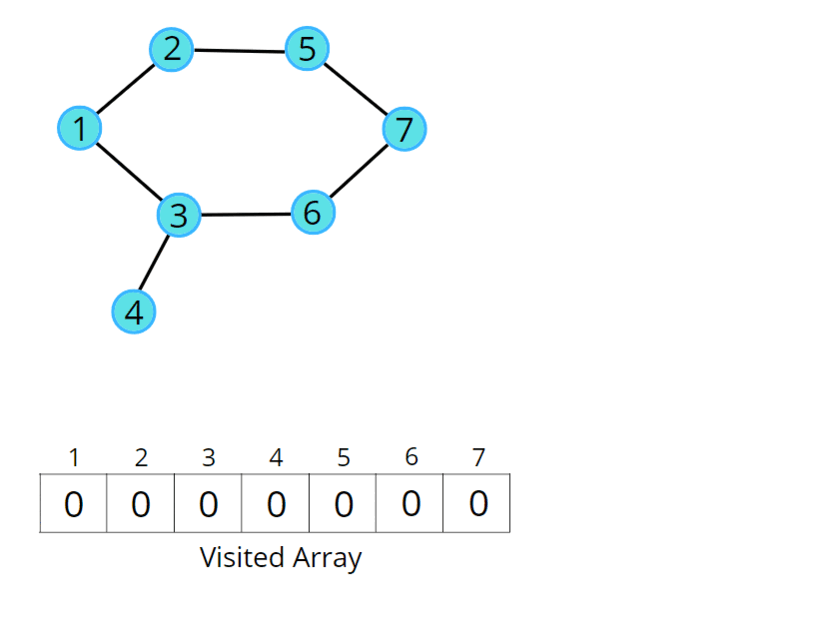Code:

## C++ Code

``````#include <bits/stdc++.h>
using namespace std;

class Solution {
private:
bool dfs(int node, int parent, int vis[], vector<int> adj[]) {
vis[node] = 1;
// visit adjacent nodes
// unvisited adjacent node
return true;
}
// visited node but not a parent node
else if(adjacentNode != parent) return true;
}
return false;
}
public:
// Function to detect cycle in an undirected graph.
bool isCycle(int V, vector<int> adj[]) {
int vis[V] = {0};
// for graph with connected components
for(int i = 0;i<V;i++) {
if(!vis[i]) {
if(dfs(i, -1, vis, adj) == true) return true;
}
}
return false;
}
};

int main() {

// V = 4, E = 2
vector<int> adj = {{}, {2}, {1, 3}, {2}};
Solution obj;
bool ans = obj.isCycle(4, adj);
if (ans)
cout << "1\n";
else
cout << "0\n";
return 0;
}
``````

Output:  0

Time Complexity: O(N + 2E) + O(N), Where N = Nodes, 2E is for total degrees as we traverse all adjacent nodes. In the case of connected components of a graph, it will take another O(N) time.

Space Complexity: O(N) + O(N) ~ O(N), Space for recursive stack space and visited array.

## Java Code

``````import java.util.*;

class Solution {
private boolean dfs(int node, int parent, int vis[], ArrayList<ArrayList<Integer>>
vis[node] = 1;
// go to all adjacent nodes
return true;
}
// if adjacent node is visited and is not its own parent node
else if(adjacentNode != parent) return true;
}
return false;
}
// Function to detect cycle in an undirected graph.
public boolean isCycle(int V, ArrayList<ArrayList<Integer>> adj) {
int vis[] = new int[V];
for(int i = 0;i<V;i++) {
if(vis[i] == 0) {
if(dfs(i, -1, vis, adj) == true) return true;
}
}
return false;
}
public static void main(String[] args)
{
ArrayList<ArrayList<Integer>> adj = new ArrayList<>();
for (int i = 0; i < 4; i++) {
}

Solution obj = new Solution();
boolean ans = obj.isCycle(4, adj);
if (ans)
System.out.println("1");
else
System.out.println("0");
}

}
``````

Output:  0

Time Complexity: O(N + 2E) + O(N), Where N = Nodes, 2E is for total degrees as we traverse all adjacent nodes. In the case of connected components of a graph, it will take another O(N) time.

Space Complexity: O(N) + O(N) ~ O(N), Space for recursive stack space and visited array.

Special thanks to Vanshika Singh Gour for contributing to this article on takeUforward. If you also wish to share your knowledge with the takeUforward fam, please check out this articleIf you want to suggest any improvement/correction in this article please mail us at [email protected]